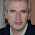## 29 may. 2012

### Mahalanobis distance with "R" (Exercice)

I have developed this exercise with Excel in another post for the same calculations , I am going to develop  it this time with  "R".
These are data of lead concentration in fish

Age Length Weight   mg/Kg

1    28    31   130.0    68.12
2    24    28   143.0   127.89
3    28    20   136.0    89.03
4    32    34   130.5    78.28
5    22    15   125.0   134.08
6    26    37   147.5   135.31
7    24    19   135.0   130.48
8    28    22   125.0    86.48
9    24    26   127.0   129.47
10   30    21   139.0    82.43
11   22    20   121.5   127.41
12   30    38   150.5    71.21
13   24    17   120.0   132.06
14   26    20   125.0    90.85

We import the data into R.
x<-read.table("C:\\lead_fish.txt",header=TRUE)
We are going to apply the Mahalanobis Distance formula:
D^2 = (x - μ)' Σ^-1 (x - μ)
We calculate ﻿μ (mean) with:
mean<-colMeans(x)
Age     Length     Weight     mg/Kg
26.28571  24.85714 132.50000 105.93571
We calculate Σ (covariance matrix (Sx)) with:
Sx<-cov(x)
> Sx
Age       Length     Weight   mg/kg
Age
9.758242   12.81319  12.07692 -72.15407
Length  12.813187  56.90110  49.11538 -70.62066
Weight  12.076923  49.11538  92.80769 -46.06962
mg/Kg  -72.154066 -70.62066 -46.06962 714.00118
The default value for the Mahalanobis function is inverted=FALSE, so the function will calculate the inverse of Sx. If we calculated appart remember to change to TRUE.
See R help:

O.K. Let´s go:
>D2<-mahalanobis(x,mean,Sx)
> D2
 5.571677 2.863499 2.686127 7.766153 2.379621 6.366793 2.135347 1.538248
 2.018812 5.143830 3.082734 5.470313 3.158651 1.818195

These are the values in the Diagonal Matrix we saw with the calculations in Excel.

#### 7 comentarios:

1.What is edad, long, peso, mg.kg ?

It would help to have just a basic understanding of what the data represents.

2.I have change the headers to english. This is data I have found in a video on Youtube with not other details.There are samples of fish giving theis age, weight, length and concentration of Lead in "ppm". I will add new exercices better documented in future posts.

3.Hi! I am interested in evaluate the statistical distance to measeure the difference between two multivariate means by the Mahalanobis distance. I am following the paper: "Statistical assesment of mean differencies between two dissolution data sets". Yi Song, Drug Information Journal, 1996.

I am struggling with the Multiple timer point dissolution. Any help could be really appreciate.
Thanks! Elba

4.Best post about Mahalanobis in "R"!!
But I have a doubt........
Is there an easy way to calculate the full Mahalanobis matrix with R, not only the main diagonal?
like:
[,1] [,2]
[1,] 5.57 -0.7 ...
[2,] -0.7 2.86....
[3,] ..................

thanks!

5.how can i create a distance matrix using Mahalanobis distance??

6.I consider the mahalanobis distance as the distance of every sample to the center of the population in a Principal Component Space. So the calculations are based on the score matrix of the samples, for a certain number on terms (PC components). This is important to see if a sample belongs to a population, or if it must be considered as an outlier. In this case I only get a MD value for every sample.
We can se a full matrix with the MD distances from a sample to the rest of the samples in the PC space, and this is the Neigbour Mahalanobis distance that you can ger with other packages.

7.How to do analysis of NIR spectra using Rstudio, how to prepare data frame and NIR spectra as input.

what is the package?